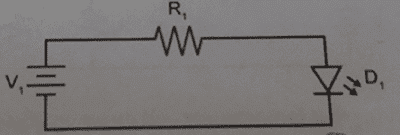# Maximum resistance and power for LED

## Homework Statement

You wish to use an LED (D1) which provides essentially zero resistance and is rated to accept either a maximum of 75 mW of power or 30 mA of current. In the following two circuits, what range values for resistor R1 will be acceptable in each case if the voltage provided by V1 is 12 V?R = V / I
V = P / I

## The Attempt at a Solution

Rmax = V / I = 12 V / 30 mA = 400 Ω.

V2 = P / I = 75 mW / 30 mA = 2.5 mV

So I need a resistor that will reduce the voltage from 12 V to 2.5 mV, requiring a voltage drop of 11.9975 V:

Rmin = V / I = 11.9975 V / 30 mA = 399.916667 Ω

Rounding to one or two significant figures (as mentioned in the instructions) would remove any sort of "range" from my answer. Furthermore, a 400V resistor would leave no energy for the LED. Is the problem in my answer or the large voltage supply?

Last edited:

gneill
Mentor
Check the units for your V2 calculation. You have mW over mA, so the "milli's" should cancel.

What does V2 represent? What's the potential drop required across the resistor taking V2 into account?

I'm not sure what criteria they want you to apply to find a "range of values" for the resistor. Perhaps they want you to select suitable values from the set of standard resistor values? See, for example, Resistors - Standard Values.

I see now that what I was doing was flawed. So 400 Ω is the minimum resistance without allowing more than 30 mA of current. I don't know what the maximum resistance would be. No matter the resistance, the voltage drop over the resistor is 12 V. The LED will never get to its maximum of 75 mW of power.

Last edited:
gneill
Mentor
I see now that what I was doing was flawed. So 400 Ω is the minimum resistance without allowing more than 30 mA of current. I don't know what the maximum resistance would be. No matter the resistance, the voltage drop over the resistor is 12 V. The LED will never get to its maximum of 75 mW of power.
Actually, the drop across the resistance will be less than 12 V. Remember V2 that you calculated before? The LED will exhibit an almost constant voltage drop due to its junction potential. LED's typically have a drop in the neighborhood of 2 or 3 V (depends upon the details of the construction and materials of the LED). So you'll want to work that into your figuring.

I'm still unsure how to select a maximum resistance without knowing something else about the LED (like a minimum current that will produce visible illumination for the application at hand).

donpacino
Gold Member
Actually, the drop across the resistance will be less than 12 V. Remember V2 that you calculated before? The LED will exhibit an almost constant voltage drop due to its junction potential. LED's typically have a drop in the neighborhood of 2 or 3 V (depends upon the details of the construction and materials of the LED). So you'll want to work that into your figuring.

I'm still unsure how to select a maximum resistance without knowing something else about the LED (like a minimum current that will produce visible illumination for the application at hand).

most LEDs are speced with a lumen/watt curve. You can either use that.

If you are not given that data and you do not when the minimum current through the LED is, then you have two options.

1. make an assumption, do some research, pick a LED and min current
2. assume the problem is simply talking about the ranges of R1 that would protect the diode, in which case R1 could be infinate

CWatters